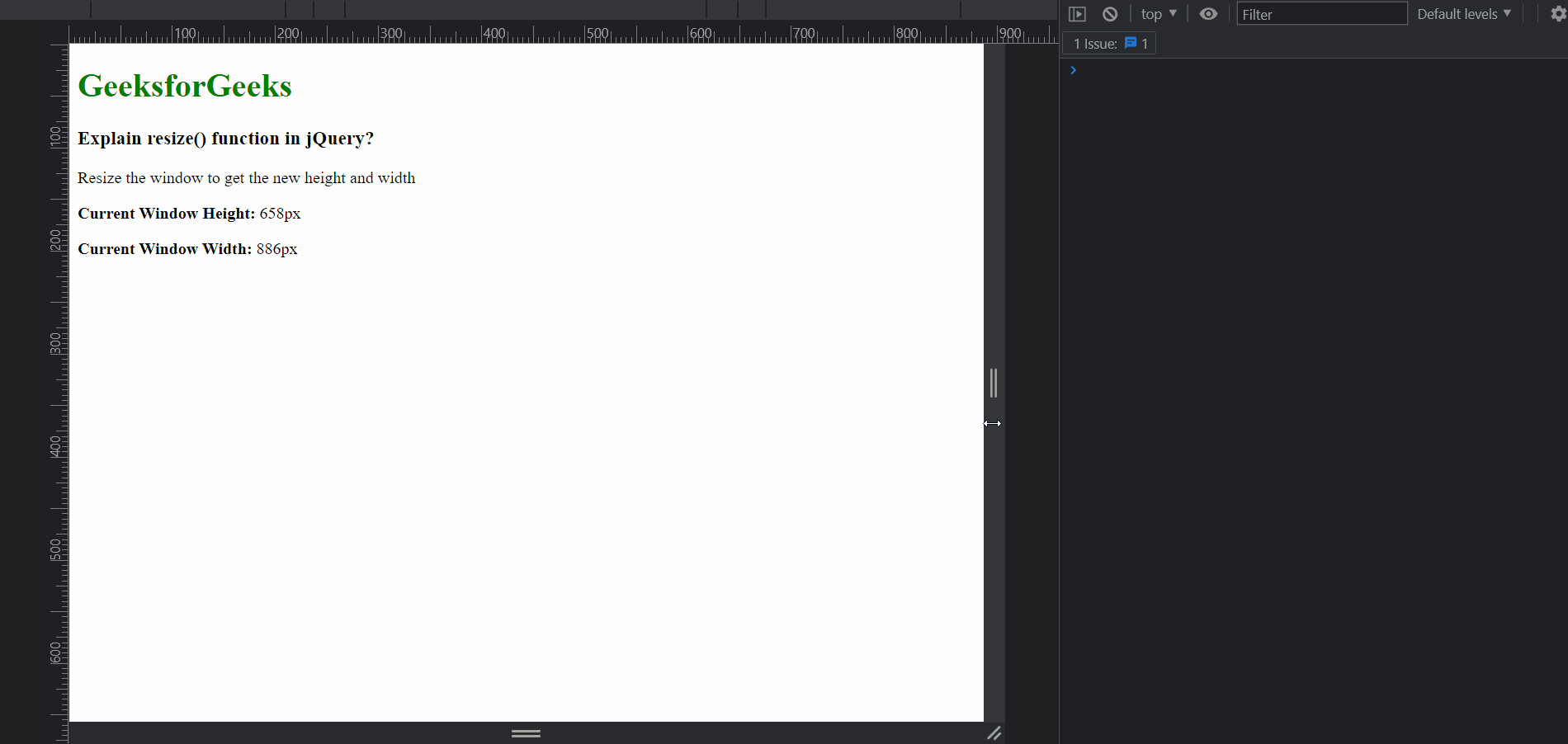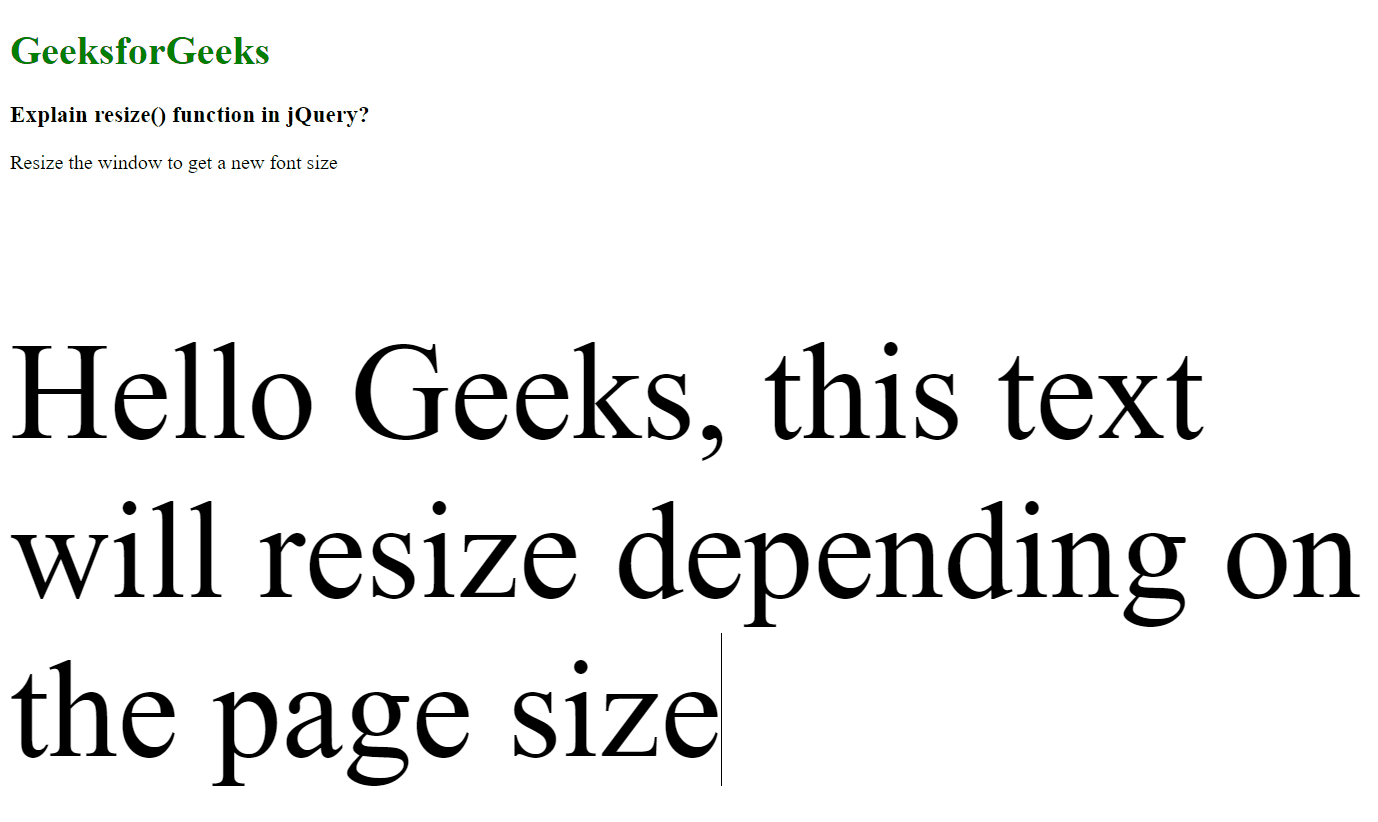# How to use resize() function in jQuery ?

• Last Updated : 23 Mar, 2022

In this article, we will learn about the resize() method which is an inbuilt method provided by jQuery. This method is used for binding an event listener to the resize event. This event is fired when the size of the browser window changes its size, either by the user or due to some other reason. This method can also be used to trigger the resize event on an element.

Note that the event may not continuously fire when the resize is taking place and may only fire in the end, hence it is not recommended to depend on the events for an exact count.

Syntax:

`\$(selector).resize( handler )`

Parameter: This method accepts a single parameter function as mentioned above and described below:

• handler: This specifies the callback handler that will be called when the resize event occurs. An optional parameter can be passed in the callback function that contains the details of the event.

The below example illustrates the resize() method in jQuery:

Example 1:

## HTML

 ` ` `<``html``> ` ` `  `<``head``> ` `    ``` `    ``<``meta` `name``=``"viewport"` `content``= ` `        ``"width=device-width, initial-scale=1.0"` `/> ` `    ``<``script` `src``= ` `        ``"https://code.jquery.com/jquery-3.6.0.min.js"``> ` `    `` ` ` ` ` `  `<``body``> ` `    ``<``h1` `style``=``"color: green;"``> ` `        ``GeeksforGeeks ` `    `` ` `     `  `    ``<``h3``>Explain resize() function in jQuery? ` ` `  `    ``<``p``> ` `        ``Resize the window to get  ` `        ``the new height and width ` `    `` ` `     `  `    ``<``p``> ` `        ``<``b``>Current Window Height: ` `        ``<``span` `id``=``"window-height"``> ` `    `` ` `     `  `    ``<``p``> ` `        ``<``b``>Current Window Width: ` `        ``<``span` `id``=``"window-width"``> ` `    `` ` ` `  `    ``<``script` `type``=``"text/javascript"``> ` `        ``\$(window).resize((eventData) => { ` `            ``console.log("Page has been resized!"); ` ` `  `            ``// Get the event type from the ` `            ``// event data available to the ` `            ``// callback function ` `            ``console.log("Event Data type", eventData.type); ` ` `  `            ``// Update the values on the page ` `            ``\$("#window-height").text(\$(window).height() + "px"); ` `            ``\$("#window-width").text(\$(window).width() + "px"); ` `        ``}); ` `    `` ` ` ` ` `  ``

Output:Example 2:

## HTML

 ` ` `<``html``> ` ` `  `<``head``> ` `    ``<``script` `src``= ` `"https://code.jquery.com/jquery-3.6.0.min.js"``> ` `    `` ` ` ` ` `  `<``body``> ` `    ``<``h1` `style``=``"color: green;"``> ` `        ``GeeksforGeeks ` `    `` ` `     `  `    ``<``h3``>Explain resize() function in jQuery? ` ` `  `    ``<``p``>Resize the window to get a new font size ` ` `  `    ``<``p` `id``=``"text"``> ` `        ``Hello Geeks, this text will resize  ` `        ``depending on the page size ` `    `` ` ` `  `    ``<``script` `type``=``"text/javascript"``> ` `        ``function resizeFont() { ` ` `  `            ``// Find the font size on the basis of ` `            ``// the current window width ` `            ``let fontSize = \$(window).width() * 0.1; ` ` `  `            ``// Update the font size ` `            ``\$("#text").css("font-size", fontSize + "px"); ` `        ``} ` `        ``resizeFont(); ` ` `  `        ``// Use the resize method with the above ` `        ``// function as the callback ` `        ``\$(window).resize(resizeFont); ` `    `` ` ` ` ` `  ``

Output:Reference: https://api.jquery.com/resize/

My Personal Notes arrow_drop_up
Recommended Articles
Page :